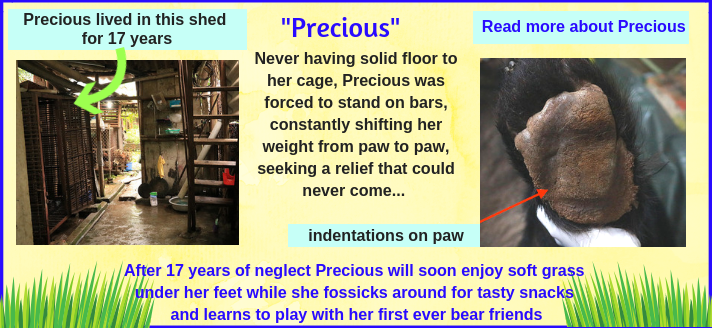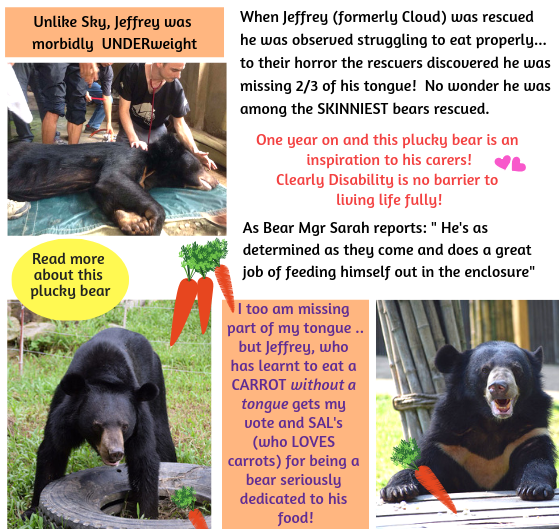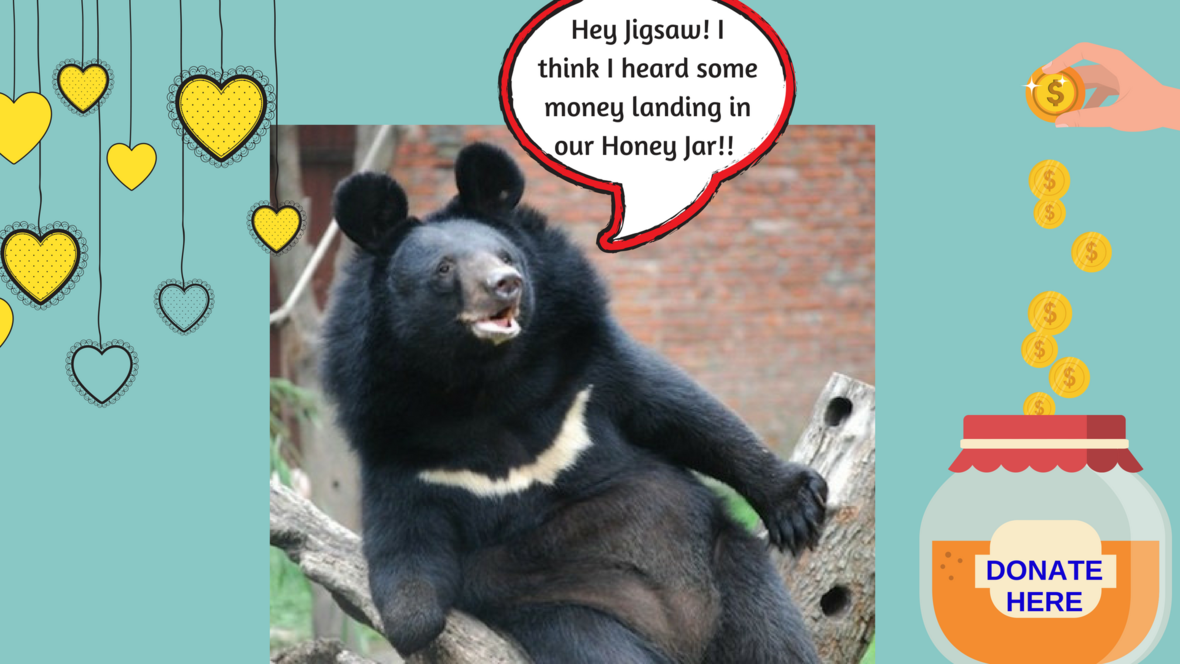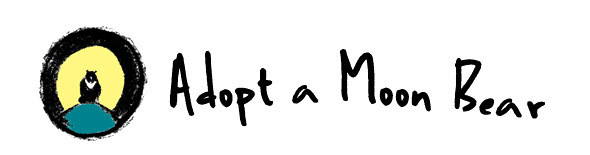table.module-1{width:53.4%;padding:0}table div table+table div table{width:53.4%;float:none;margin-left:auto;margin-right:auto;padding:0}table div table+table div table a{border:0 none;text-decoration:none}table div table+table div table img{width:100%!important;border:0 none;text-decoration:none}table div table+table div table td{width:100%;padding:0}/* styles */
 table div table+table+table div table{width:100%;padding:0}table div table+table+table div table img{width:96.23%;padding:0;float:none}table div table+table+table div table td{width:100%;padding:0 1.88% 18px}/* styles */## Hi Everyone, it’s your bear, Jigsaw, here and the good news keeps rolling in!ONLY 13 PIECES LEFT TO DONATE!! GET ONE HERE!

## Super happy, too, because the 5 bears rescued in August are already relishing their new lives!ENJOY A SHORT VIDEO OF THESE BEARS PLAYING IN THEIR DENS

## Since August another two bears have been "welcomed home" here in Vietnam: After 13 years of solitude Sky now has a long road to a healthy recovery ahead of her.WATCH A SHORT YOUTUBE OF A HAPPY SKY, PLAYING IN HER RECOVERY DEN. SOON SHE WILL BE OUTSIDE

## After enduring 17 years of suffering Precious is yet to discover how good her life is going to be!READ ABOUT THE CARE PRECIOUS IS RECEIVING

## And Precious also had no idea of how extra special she would be... she's a Milestone Bear!CLICK HERE TO READ MORE ABOUT PLANNING FOR THE FUTURE
 table div table+table+table+table+table+table+table+table+table+table+table+table+table+table div table{width:100%;padding:0}table div table+table+table+table+table+table+table+table+table+table+table+table+table+table div table img{width:96.23%;padding:0;float:none}table div table+table+table+table+table+table+table+table+table+table+table+table+table+table div table td{width:100%;padding:0 1.88% 18px}/* styles */## Why we care.... Because even a bear with 2/3 of his tongue torn out can still develop a big appetite for life..READ MORE ABOUT A VERY PLUCKY BEAR WHO LOVES LIFE!
 table div table+table+table+table+table+table+table+table+table+table+table+table+table+table+table+table+table div table{width:100%;padding:0}table div table+table+table+table+table+table+table+table+table+table+table+table+table+table+table+table+table div table img{width:96.23%;padding:0;float:none}table div table+table+table+table+table+table+table+table+table+table+table+table+table+table+table+table+table div table td{width:100%;padding:0 1.88% 18px}/* styles */## Oops! Almost forgot to show you my puzzle.... As you can see, I am SO CLOSE to being finished - only 13 pieces!! Will you help me over the finish line?

 table div table+table+table+table+table+table+table+table+table+table+table+table+table+table+table+table+table+table+table div table td,table.module-18{width:100%;padding:0}table div table+table+table+table+table+table+table+table+table+table+table+table+table+table+table+table+table+table+table div table{width:100%;float:none;margin-left:auto;margin-right:auto;padding:0}table div table+table+table+table+table+table+table+table+table+table+table+table+table+table+table+table+table+table+table div table a{border:0 none;text-decoration:none}table div table+table+table+table+table+table+table+table+table+table+table+table+table+table+table+table+table+table+table div table img{width:100%!important;border:0 none;text-decoration:none}/* styles */
 table.module-19{width:60.38%;padding:0}table div table+table+table+table+table+table+table+table+table+table+table+table+table+table+table+table+table+table+table+table div table{width:60.38%;float:none;margin-left:auto;margin-right:auto;padding:0}table div table+table+table+table+table+table+table+table+table+table+table+table+table+table+table+table+table+table+table+table div table a{border:0 none;text-decoration:none}table div table+table+table+table+table+table+table+table+table+table+table+table+table+table+table+table+table+table+table+table div table img{width:100%!important;border:0 none;text-decoration:none}table div table+table+table+table+table+table+table+table+table+table+table+table+table+table+table+table+table+table+table+table div table td{width:100%;padding:0}/* styles */CLICK ON JINGLE'S HONEY JAR TO DONATE ! THANKS!table div table+table+table+table+table+table+table+table+table+table+table+table+table+table+table+table+table+table+table+table+table+table+table+table div table{width:100%;padding:0}table div table+table+table+table+table+table+table+table+table+table+table+table+table+table+table+table+table+table+table+table+table+table+table+table div table img{width:96.23%;padding:0;float:none}table div table+table+table+table+table+table+table+table+table+table+table+table+table+table+table+table+table+table+table+table+table+table+table+table div table td{width:100%;padding:0 1.88% 18px}/* styles */